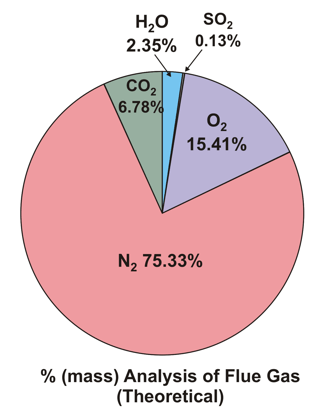Operational Theory

Combustion Air and Products of Combustion

 ****JavaScript based drop down DHTML menu generated by NavStudio. (OpenCube Inc. - http://www.opencube.com)****

A typical marine heavy fuel oil consists of 85% Carbon, 12% Hydrogen and 3% Sulphur by mass. (here, the tiny amounts of sodium, vanadium, heavy metals, cat fines, ashes etc. are being ignored)Breakdown of 1kg of Fuel

Using simple chemistry it is fairly easy to calculate the minimum mass of air that would be required for complete combustion of the fuel.

Carbon burns in oxygen to produce carbon dioxide

C + O2 → CO2

If the molecular masses are now inserted;

12 + 32 → 44

This actually means that if we had 12kg of carbon, it would take 32 kg of oxygen to burn it  to 44 Kg of carbon dioxide

However in our 1 kg of fuel we have only 0.85 kg carbon, so our sum becomes

0.85 + 2.27 → 3.12

So we need 2.27kg of oxygen to burn the carbon in our 1kg of fuel.

Hydrogen burns in oxygen to produce water

2H2 + O2 → 2H2O

If the molecular masses are now inserted;

4 + 32 → 36

This actually means that if we had 4kg of hydrogen, it would take 32 kg of oxygen to burn it  to 36 Kg of water

However in our 1 kg of fuel we have only 0.12 kg hydrogen, so our sum becomes

0.12 + 0.96 → 1.08

So we need 0.96kg of oxygen to burn the hydrogen in our 1kg of fuel

Sulphur burns in oxygen to produce sulphur dioxide

S + O2 → SO2

If the molecular masses are now inserted;

32 + 32 → 64

This actually means that if we had 32kg of sulphur, it would take 32 kg of oxygen to burn it  to 64 kg of sulphur dioxide

However in our 1 kg of fuel we have only 0.03 kg sulphur, so our sum becomes

0.03 + 0.03 → 0.06

So we need 0.03kg of oxygen to burn the sulphur in our 1kg of fuel

If the oxygen to burn the carbon, hydrogen and sulphur is now added up it comes to 3.26kg, which is the mass of oxygen required to burn 1 kg of the fuel completely.

Air contains 23% oxygen, 77% Nitrogen by mass (these are the two major constituents of air; The other gases are present in such small quantities- yes, even carbon dioxide,- that they can be ignored.)

The mass of air that must be supplied to contain the required oxygen is: 3.26 ÷ 0.23 = 14.17kg. However in a diesel engine, to scavenge the cylinder and to ensure complete combustion, we always supply more than the minimum required air (excess air), up to about 3.5 times as much (50kg air/kg fuel.) However for this calculation we will assume we are supplying 45kg air/kg fuel which is 30.83kg of excess air.

The mass of our products of combustion can be now calculated, and they must add up to 46 kg (45 kg air + 1 kg fuel)

 CO2     (from equation above)           3.12kg H2O     (from equation above)           1.08kg SO2      (from equation above)           0.06kg N2         (77% of air supplied)          34.65kg O2     (from the excess air supplied)    7.09kg   Total                                                46.00kgDownload this spreadsheet and alter the fuel constituent ratios and the excess air and see the results.

To be be absolutely accurate, the small amount of cylinder lubricant which will also be burnt should be taken into account. However, if the engine is being operated efficiently this amount is very small (about 3 grams, for every kg of fuel burnt)

In Practice, the air entering the engine will contain some moisture, and so this must be taken into account. A very small amount of nitrogen will combine with oxygen at the high temperatures inside the cylinder and form oxides of Nitrogen (known as NOx). Even with correct combustion, a small amount of carbon will only partially burn to carbon monoxide. In a well maintained diesel engine this will be approximately 60ppm (0.003kg for every kg of fuel burnt), and there will also be a small amount of partially burnt and unburnt hydrocarbons and particulates in the exhaust.

The temperature of the intake air will vary according to climate. However if it is assumed that the air in the engine room is 30°C with a relative humidity of 50%, then it will contain about 13.35g of water/kg air. If the air is compressed in the turbocharger to 2.5 bar (gauge) and then cooled to 45° in the air cooler then it's relative humidity rises to 77% with a dewpoint of 40°. This means that the water will remain in the air and pass through the engine. The total water will now increase by 45 (kg of air) × 13.35 = 600 grams or 0.6 kg.

A useful calculator which calculates the mass of water/kg air, dewpoint, relative and absolute humidity, for various pressure and temperature can be found at:

The NOx produced in engines installed since 2000 is limited and dependant on engine speed.

Limits:

17g/kWh engine revs below 130

45×n-0.2 g/kWh engine revs between 130 and 2000

9.8g/kWh where engine revs are above 2000

This means that on a slow speed 2 stroke engine the NOx produced is less than 0.1kg for every kg of fuel burnt.

As From 1st January 2011 the limits change;:

For engines less than 130RPM: 14.4g/kWh

For Engines between 130 and 2000 rpm: 44 × n-0.23 g/kWh

For engines above 2000 rpm: 7.7g/kWh

After 2016 they change again to:

For engines less than 130RPM: 3.4g/kWh

For Engines between 130 and 2000 rpm: 9 × n-0.2 g/kWh

For engines above 2000 rpm: 2.0g/kWh